# Visualizing The Volume of a Cylinder

Visual number talk prompts to build on the conceptual understanding of area of a circle to emerge the formula for volume of a cylinder.

## In This Set of Math Visual Prompts…

Students will investigate the relationships between the area of the base, the height, and the volume of a cylinder.

## Intentionality…

The purpose of this set of visual number talk activities is to reinforce the concepts students would learn during the problem based math lesson called Pop Goes The Volume [Day 1]

Some of the big ideas that will likely emerge in this visual number talk include:

• The volume of a 3-dimensional figure can be found by determining the number of cubic units that can be contained within the figure;
• The volume of a prism can be determined by finding the number of cubic units required to cover the base and multiply by the number of layers (i.e.: the height); and,
• Varying the width of the base of a cylinder (i.e.: the diameter) has a greater impact on the volume of a cylinder than varying the height of the cylinder.

## Preparing to Facilitate This Number Talk

The following sequence of related problems provides an opportunity for students to build on their learning from a volume investigation by working through the volume of a cylinder with missing dimensions or limited information. This provides a context for students to investigate different approaches for determining the volume of a cylinder by grounding their thinking in the relationship between the area of the base and the height of a cylinder. In the previous lesson, we explored the context for comparing two cylinders in order to determine which one has the greatest volume. This context was helpful for building conceptual understanding supporting students in their understanding of Pi, π, and generalizing for how to calculate the volume of a cylinder.

## Visual Math Talk Prompt #1

Play the following visual math talk prompt and be prepared to pause where indicated:

Prompt:

Without using a calculator, what might the approximate volume of this cylinder be?

How might you approach determining the volume of this cylinder?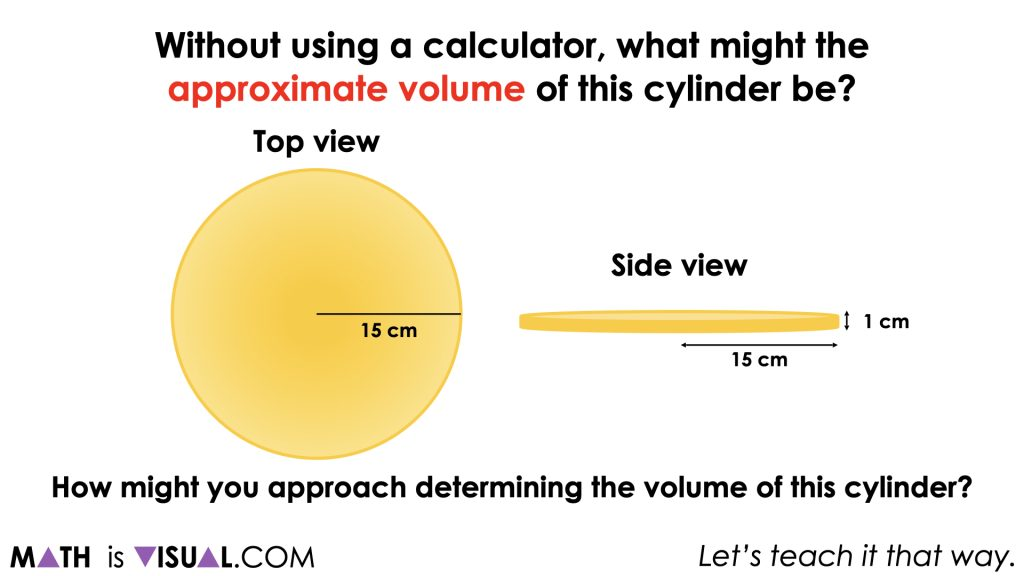The purpose here is for students to rely on their understanding of the area of a circle (needed for calculating the area of the base) prior to adding in a height beyond 1 ‘layer’ of cubic centimetres.

With the image projected, give students 30 seconds to 1 minute to reflect on the question. Then students will do a quick turn and talk to share their thinking with a classmate.

After a reasonable amount of time, invite students to share their approximations.

Be sure to record student thinking in a space (eg., whiteboard) visible to all students. This honours the contributions of all students.

The intention of this math talk is to get students using the relationship of the diameter of a circle to its radius (Pi, π) to determine an approximate circumference. Since the radius is 15 cm, the diameter of the circular base is 30 cm and we can use our understanding that the circumference of a circle is approximately 3 “and a bit” (Pi, π) times as long as the diameter.

A reasonable estimate for the circumference would be between 90 cm and 95 cm.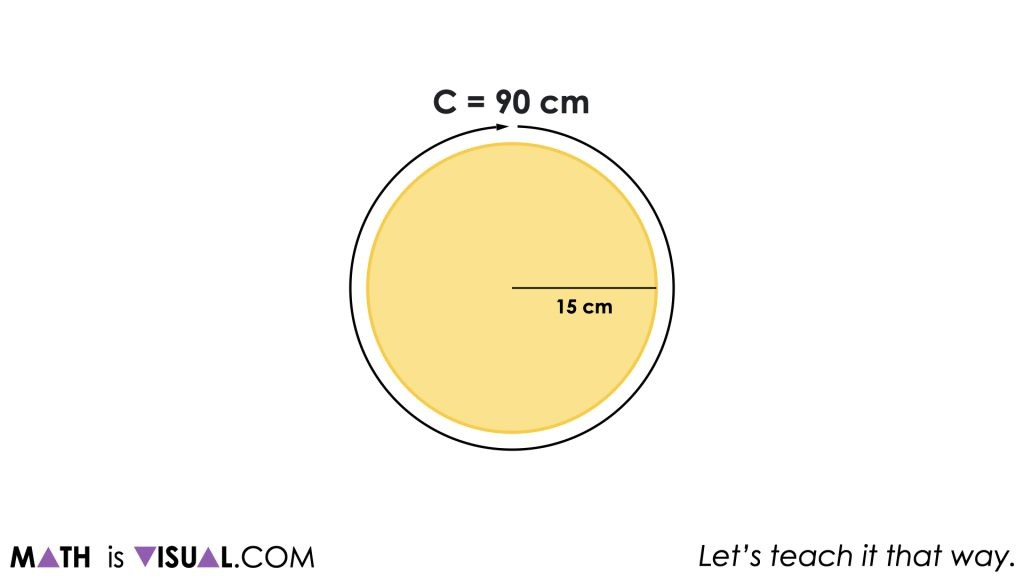With this, we hope that students are able to visualize (or draw) that the area of a circle can be found by decomposing the circle and recomposing into a parallelogram or rectangle as we explored in the Going In Circles unit. Through that unit, we learned that the width would be equivalent to the radius and the width would be equivalent to half of the circumference.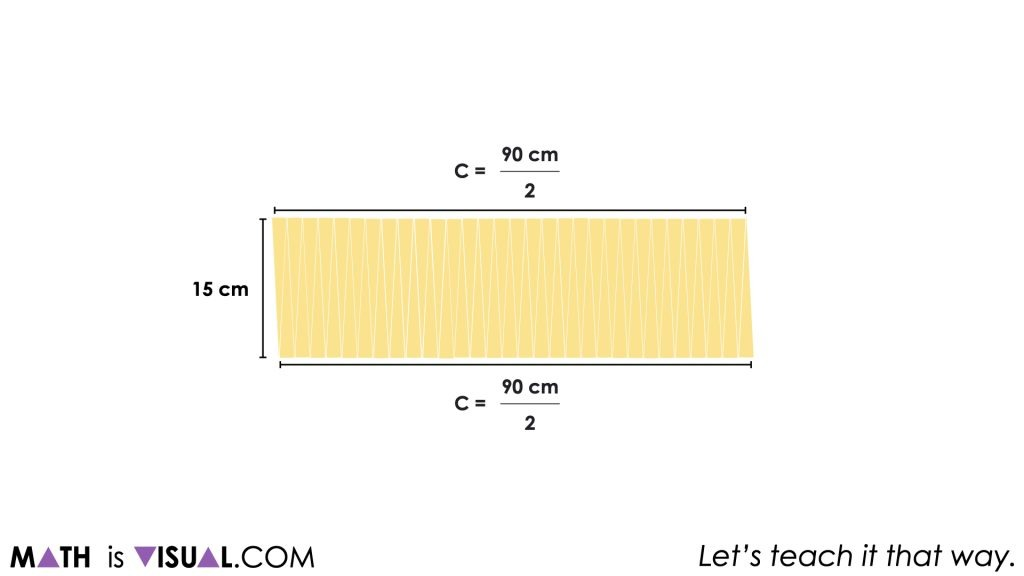There may be some students who recall the formula for area of a circle as π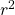and that is completely fine as well. We still encourage those students to determine the result without the use of a calculator.

Regardless of which approach students take to solve for the area of the base, a common strategy you might see students use is partial products where the area of a rectangle is partitioned into two or more smaller product parts and then recomposed afterwards.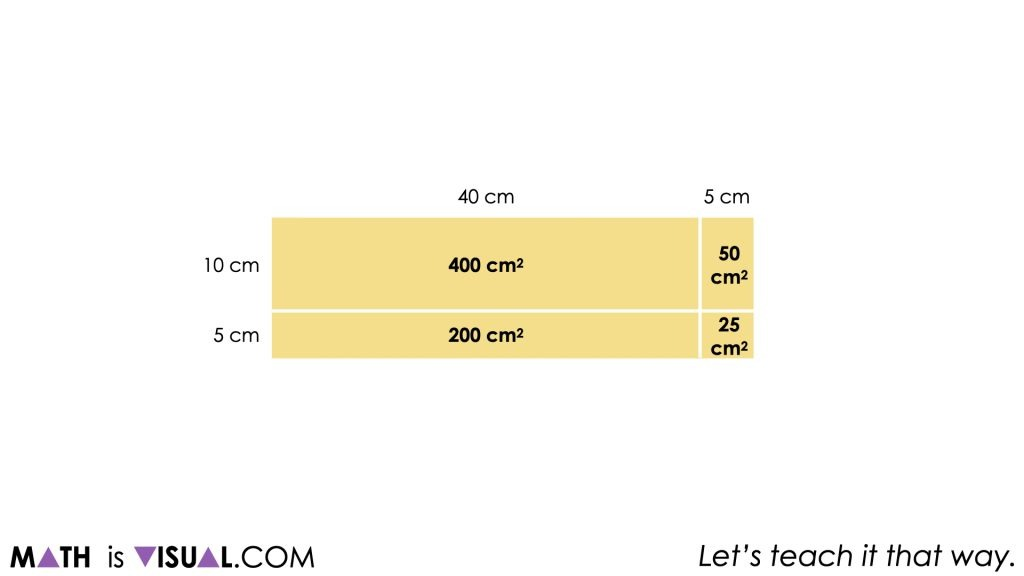Visual Math Talk Prompt #1 serves as a means to recall prior knowledge regarding the area of a circle and hopes to emerge the idea that a cylinder with a height of only 1 unit simply transforms the number of ‘squares’ we calculated for the area into the same number of ‘cubes’.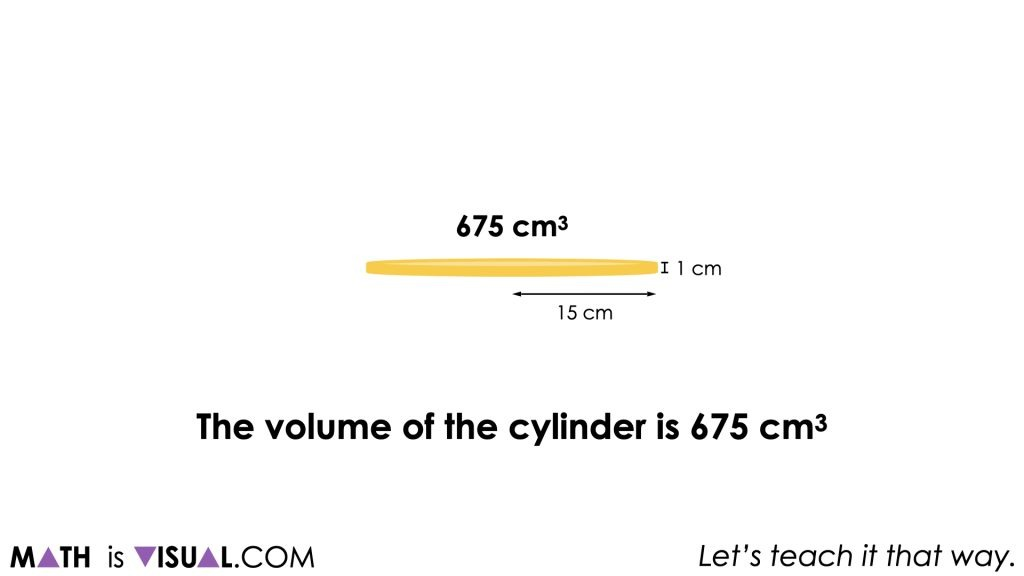Therefore, the area of the base for this one layer cylinder is 675 square centimetres and has a volume of 675 cubic centimeters.

## Visual Math Talk Prompt #2

Consider utilizing the following visual math talk prompt and be prepared to pause when indicated:

The following prompt will appear on the screen:

Without using a calculator, what might the approximate volume of this cylinder be?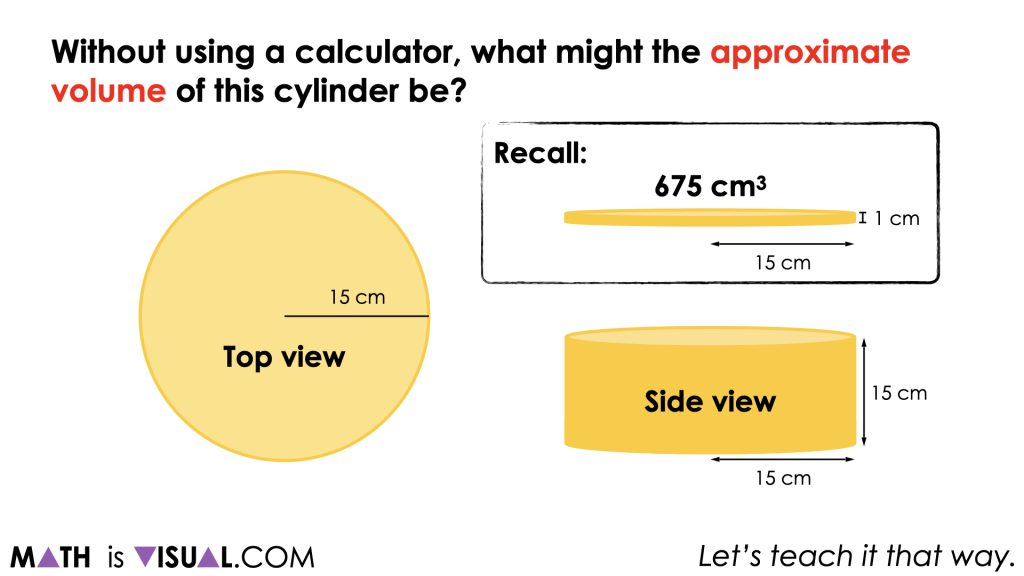With the image projected, give students 30 seconds to 1 minute to reflect on the question. Then students will do a quick turn and talk to share their thinking with a classmate.

After a reasonable amount of time, invite students to share their approximations.

Be sure to record student thinking in a space (eg., whiteboard) visible to all students. This honours the contributions of all students.

Some students may immediately recognize that this cylinder has the same base as the cylinder from the previous Visual Math Talk Prompt, however the height has increased from 1 cm to 15 cm tall.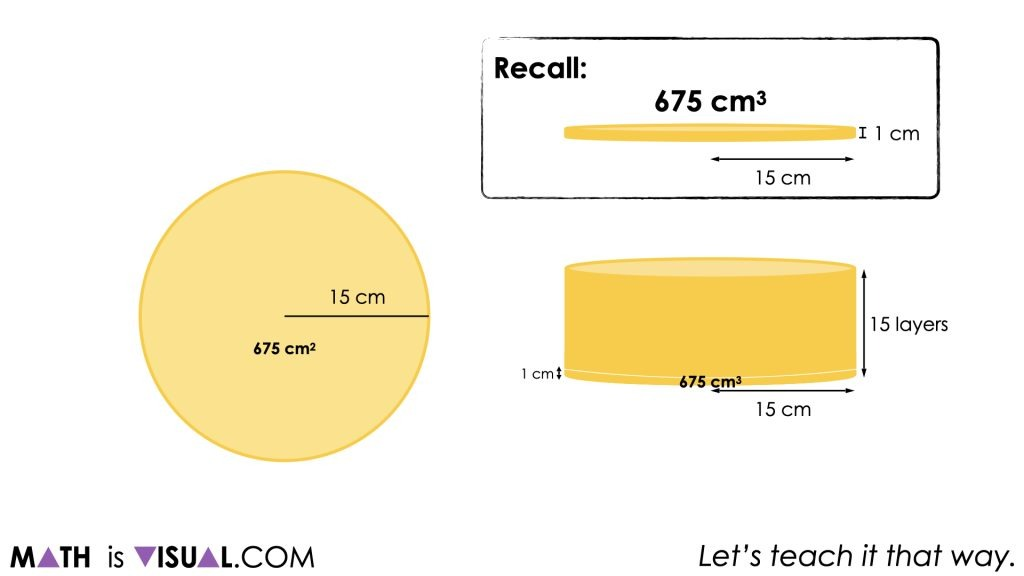In other words, this cylinder has 15 times as many “layers” as the previous cylinder. Therefore, students who are additive thinkers can add 675 cubic centimeters repeatedly until they reach the 15th layer. Other students might choose to use a doubling strategy; doubling the volume of 1 layer to the volume of 2 layers; from 2 layers to 4 layers; 4 layers to 8 layers; and so on.

A worthwhile strategy to share and make connections to would be leveraging partial products where we determine the volume of say 10 layers and 5 layers before finding the sum.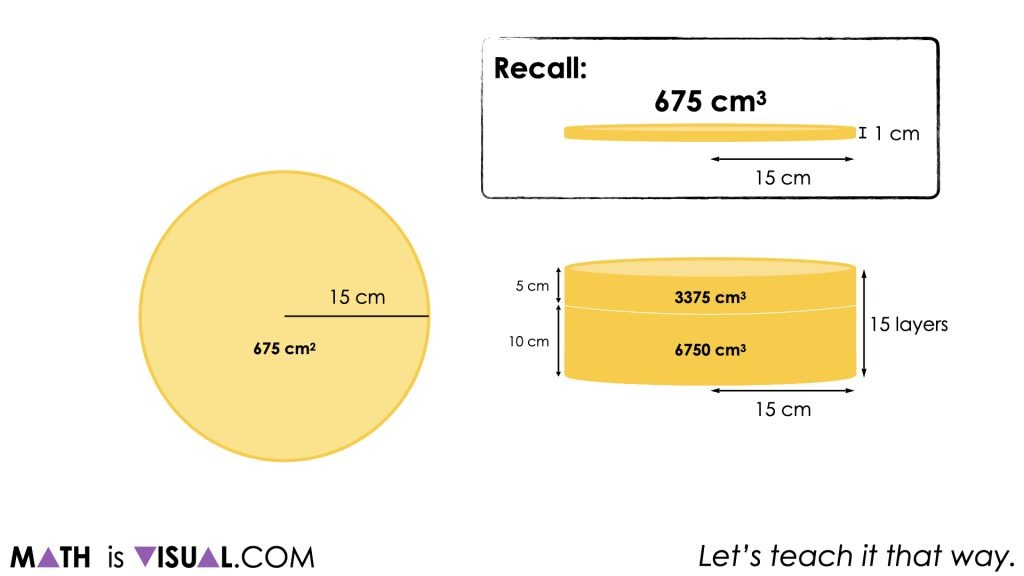It should be noted that different approximations used for the circumference and area of the base will impact the actual volume of the cylinder.

## Want to Explore These Concepts & Skills Further?

Two (2) additional number talk prompts are available in Day 2 of the Pop Goes The Volume problem based math unit that you can dive into now.

Why not start from the beginning of this contextual 5-day unit of real world lessons from the Make Math Moments Problem Based Units page.

Did you use this in your classroom or at home? How’d it go? Post in the comments!

Math IS Visual. Let’s teach it that way.